Python环境配置教程

================

# 一、准备相应的材料

Anaconda3-2021.05-Windows-x86_64.exe

Anaconda的安装包

Miniconda3-py39_4.9.2-Windows-x86_64.exe

pycharm-community-2021.1.3.exe

PyCharm安装包

Python代码的编辑器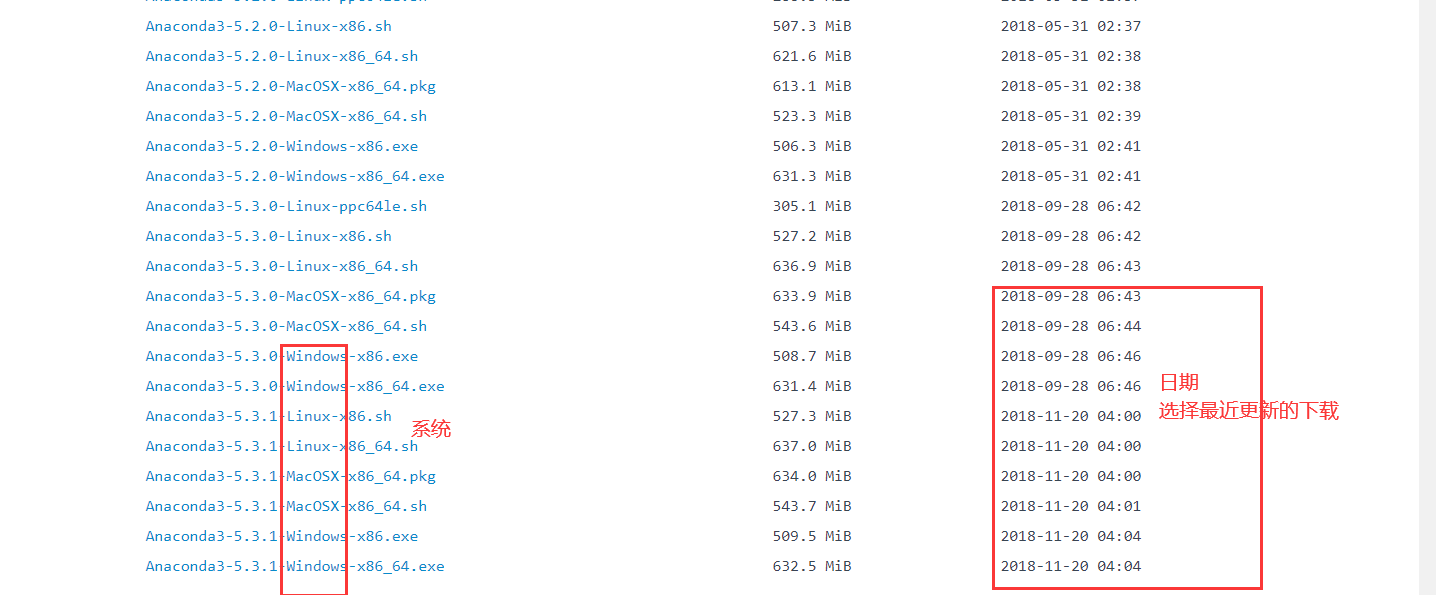PyCharm下载时选择Community 版本为免费版本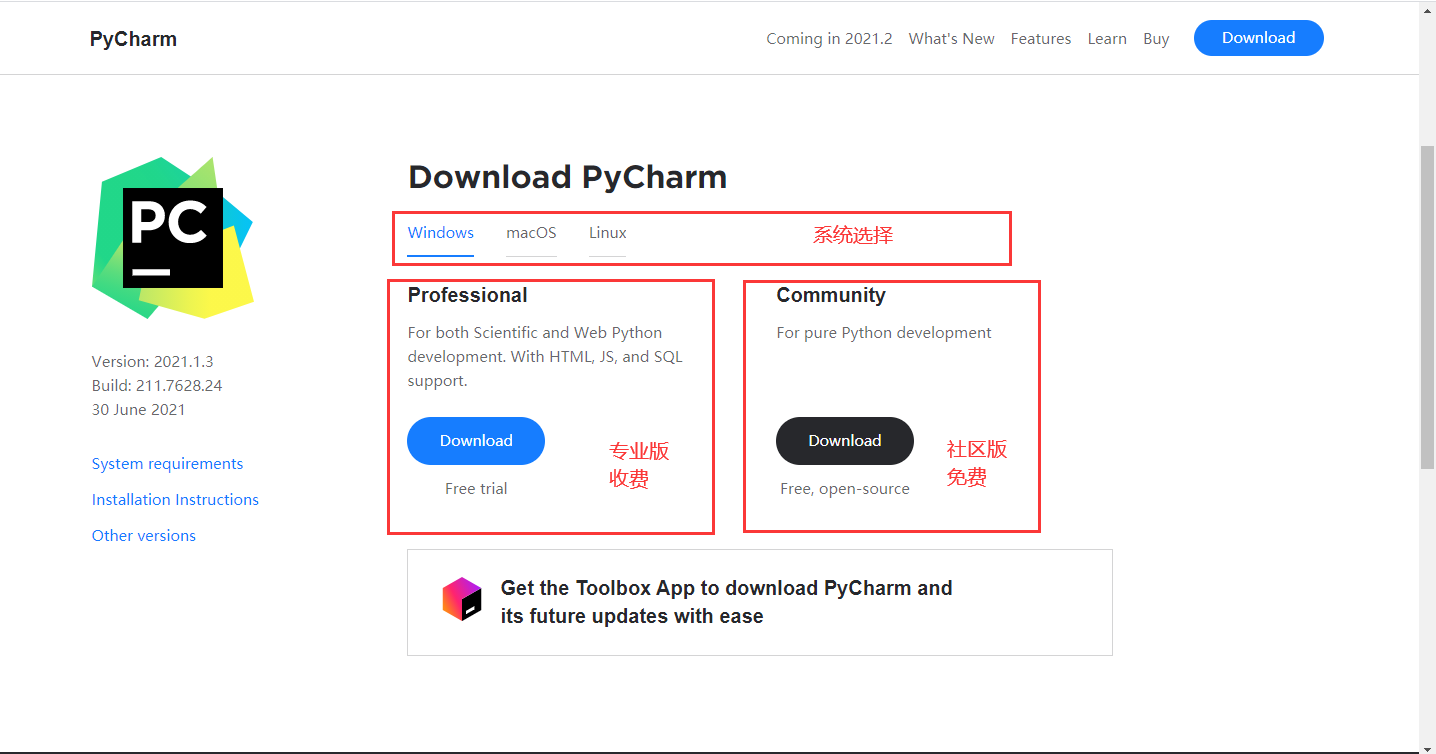# 二、安装Anaconda

1. 双击打开Anaconda3-2021.05-Windows-x86_64.exe文件（电脑配置较差的可以换成Miniconda3-py39_4.9.2-Windows-x86_64.exe，两者在基本功能上没有太大区别，二者选其一即可）

2. 出现安装界面，按照以下流程选择安装。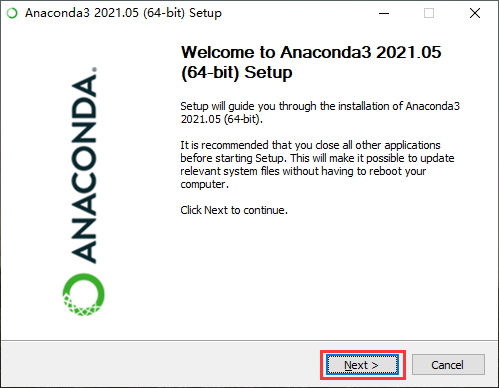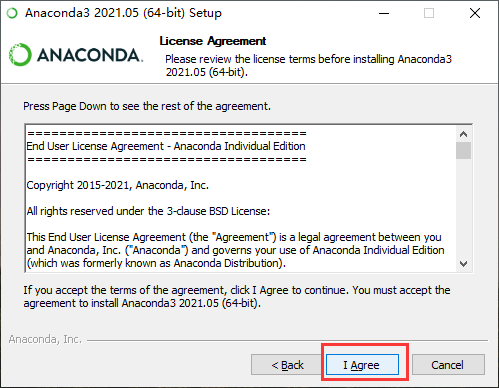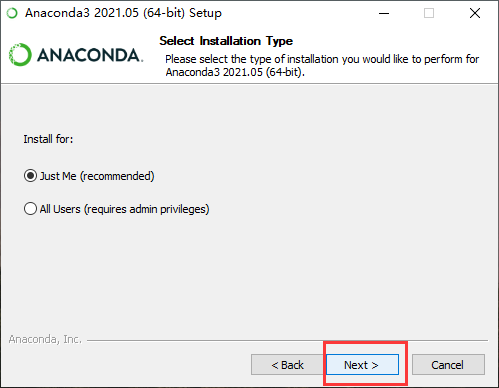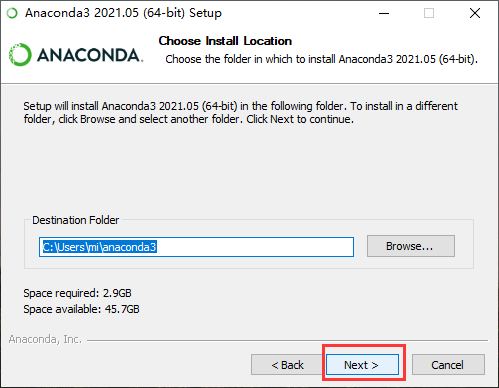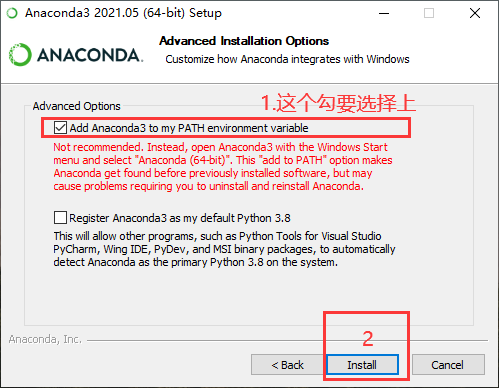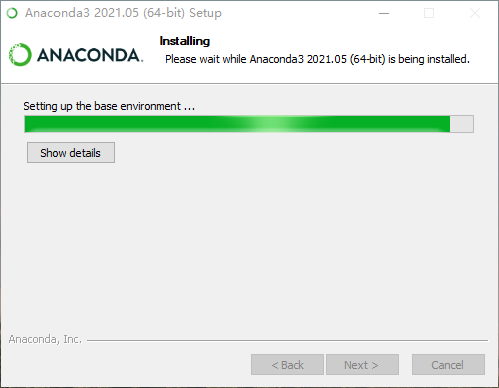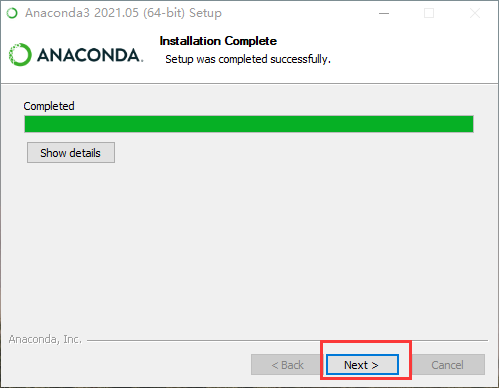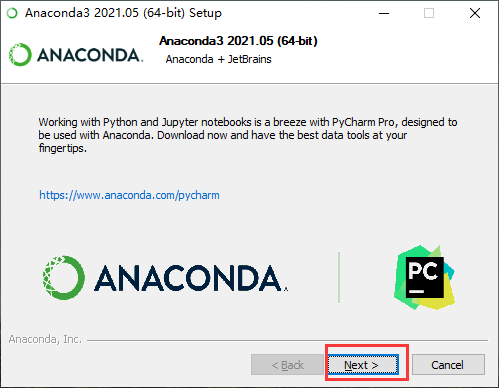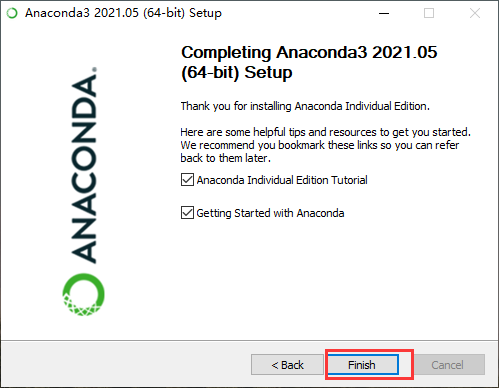Anaconda安装完成

# 三、安装PyCharm

1. 双击打开Anaconda3-2021.05-Windows-x86_64.exe文件。

2. 出现安装界面，按照以下流程选择安装。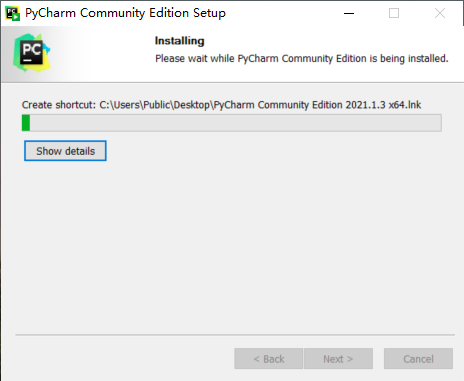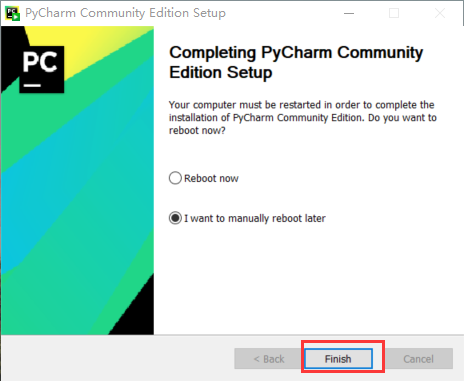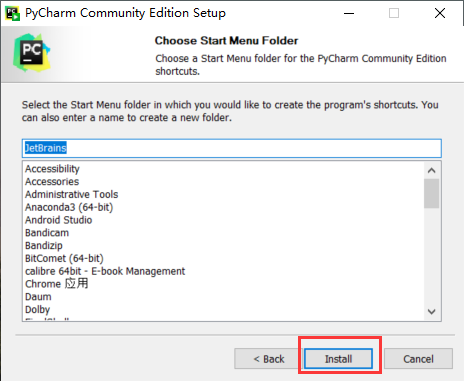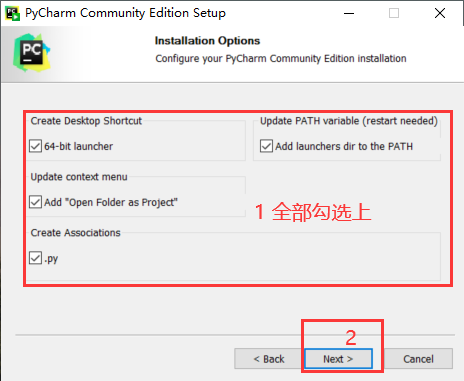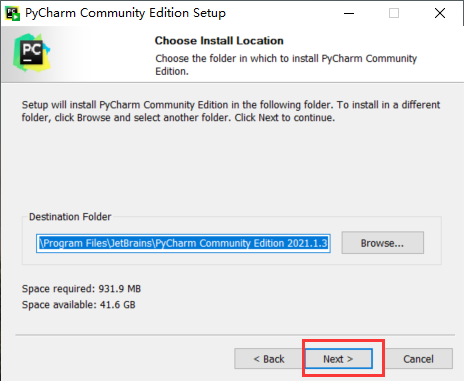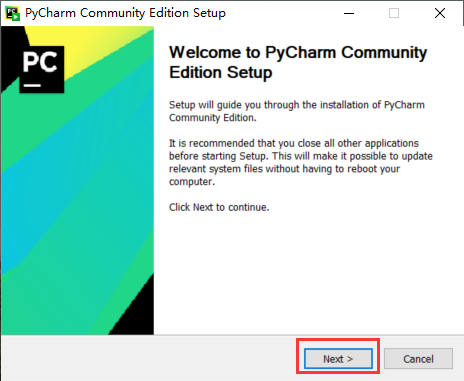# 四、配置环境

1. 在开始任务栏打开刚才安装的Anaconda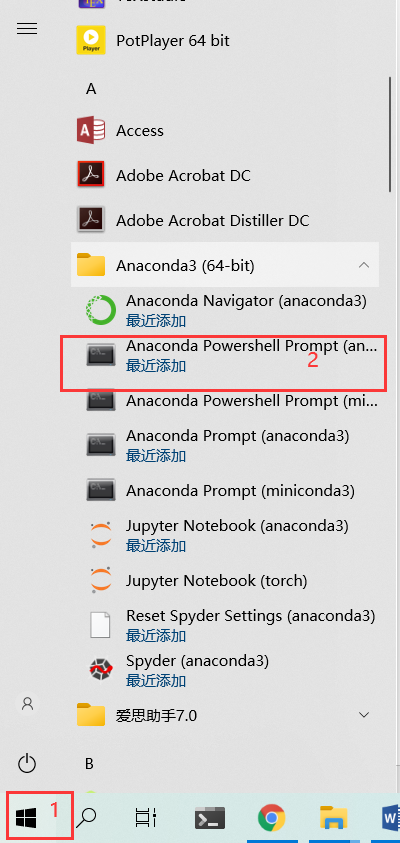出现如下界面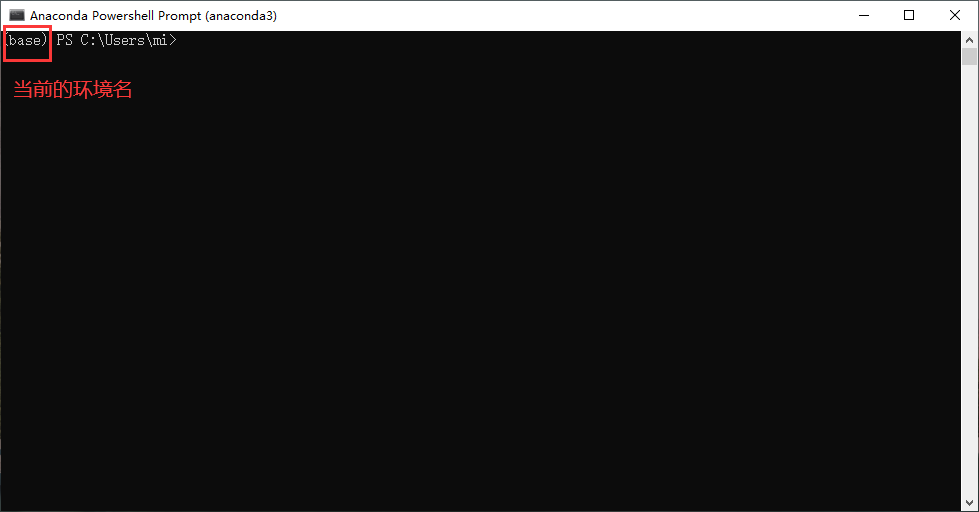1. 创建一个新的环境，名为demo（名字可以任意起，给环境起标记作用）。

操作如下：

（1）输入:

`````` conda create -n demo python=3.8
``````

（2） 按Enter键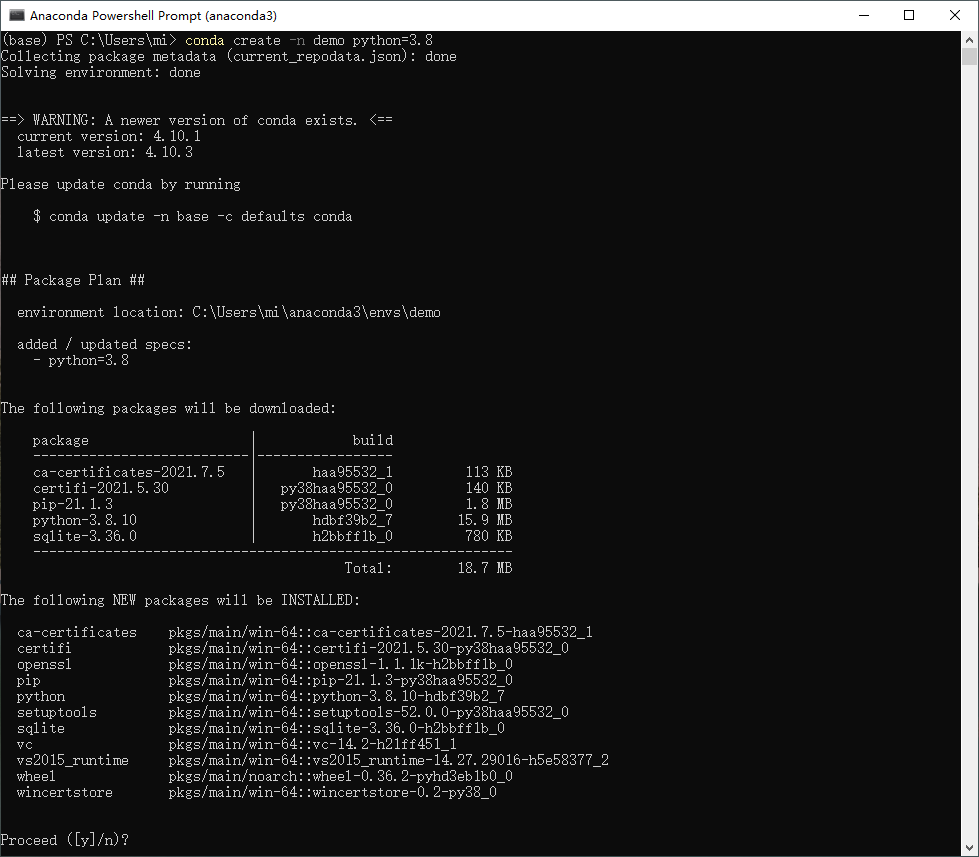（1）输入：

``````y
``````

（2） 按Enter键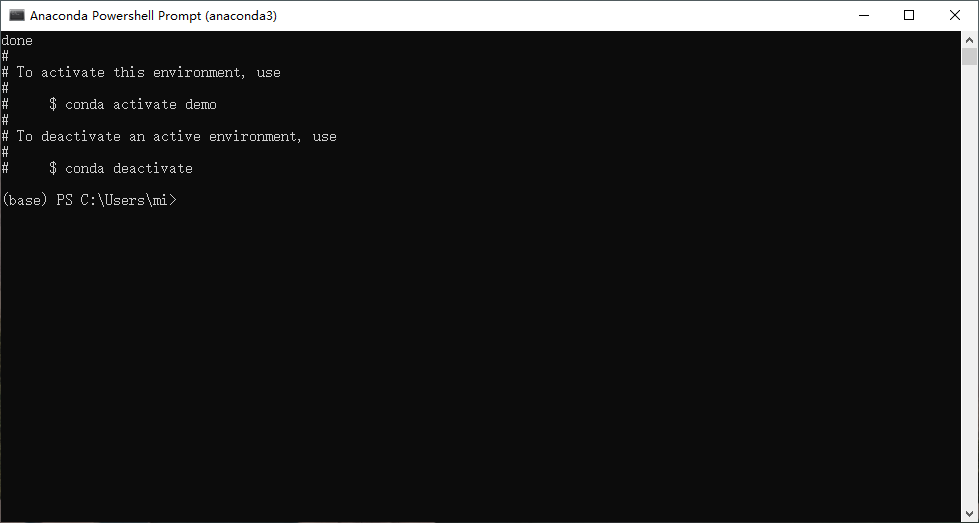1. 打开PyCharm，创建新项目，配置新建环境。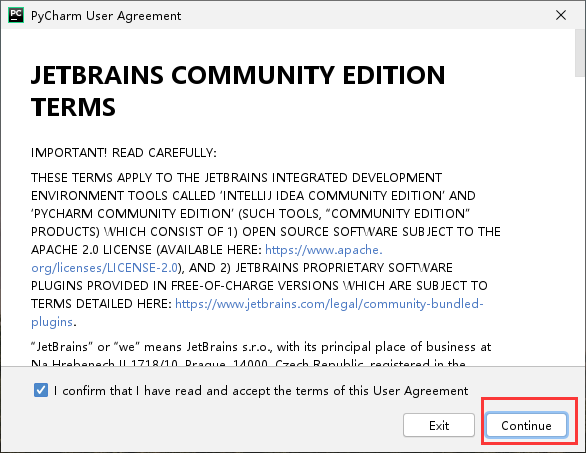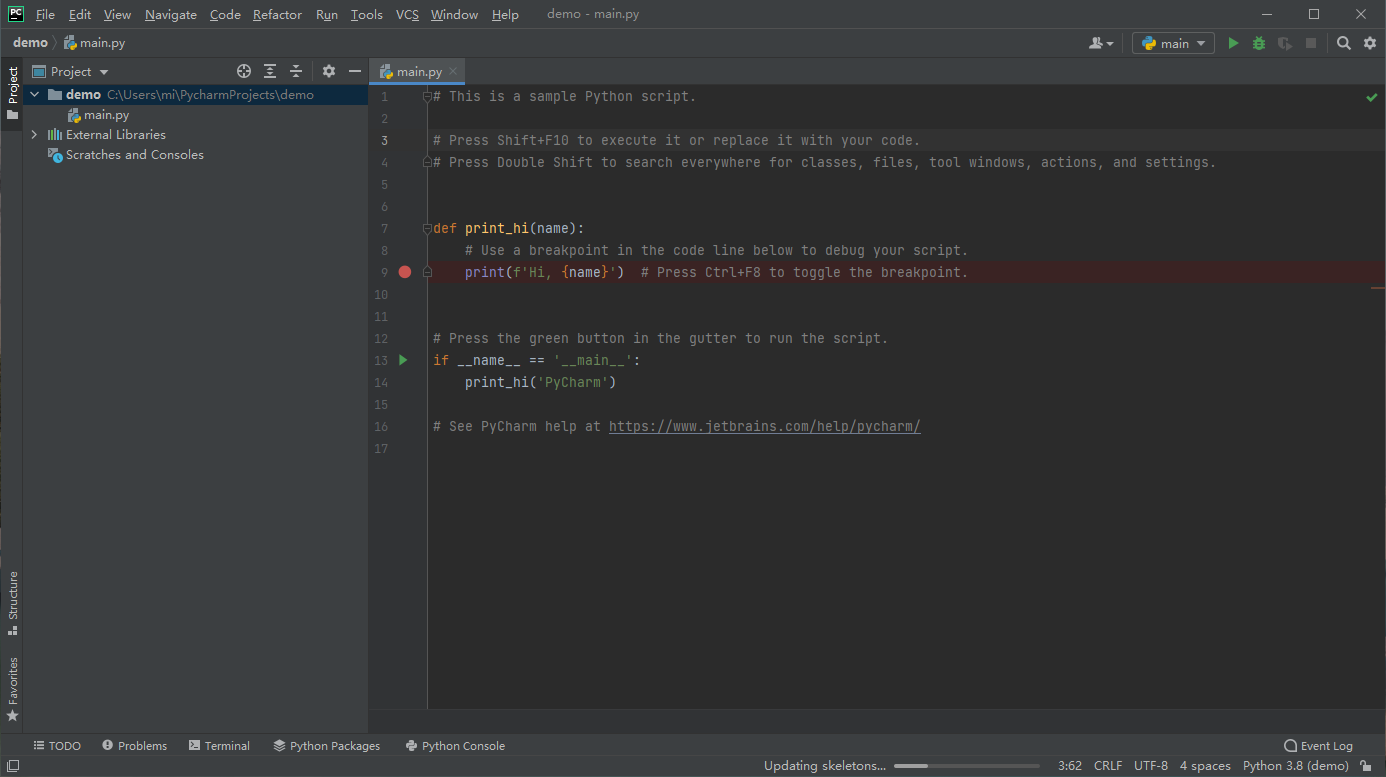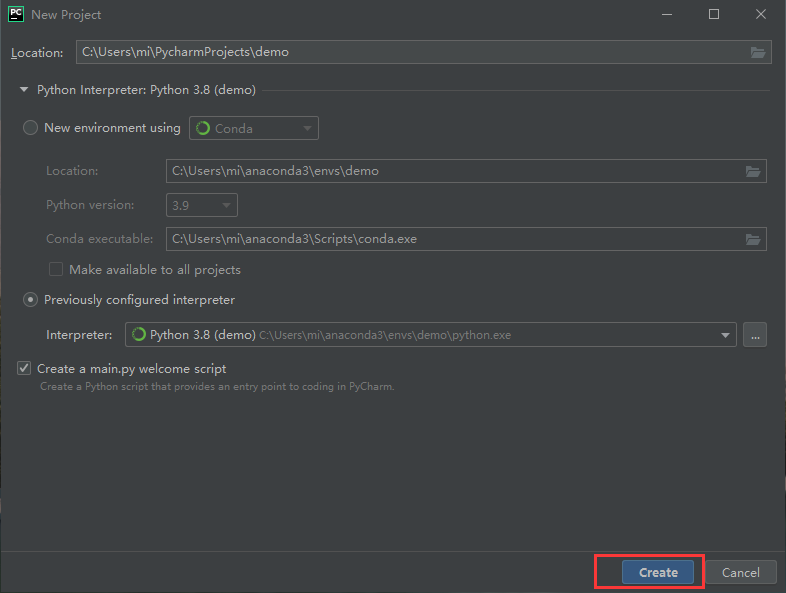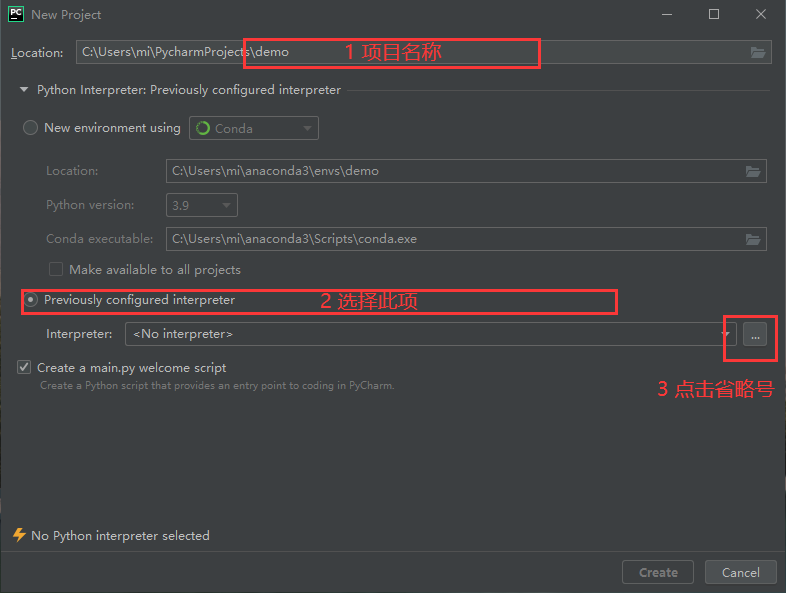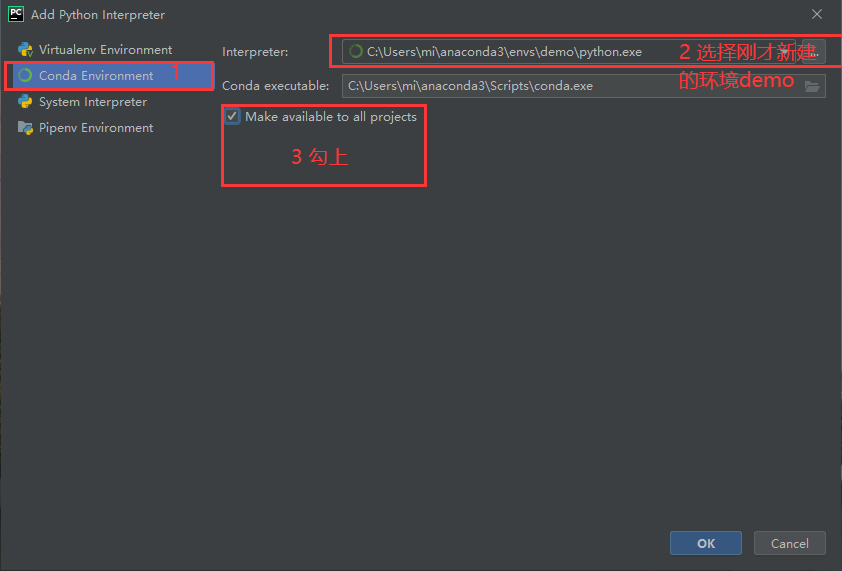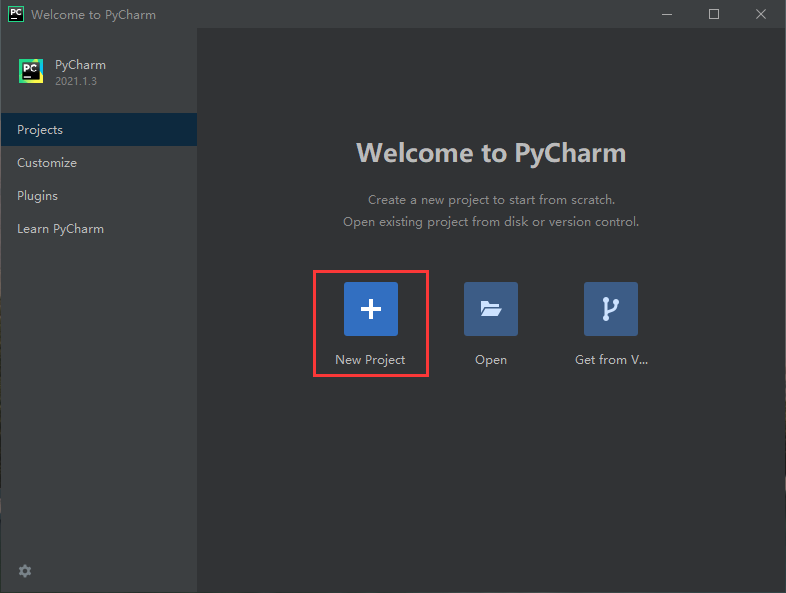1. 运行Python文件。PyCharm功能区分布大致如下。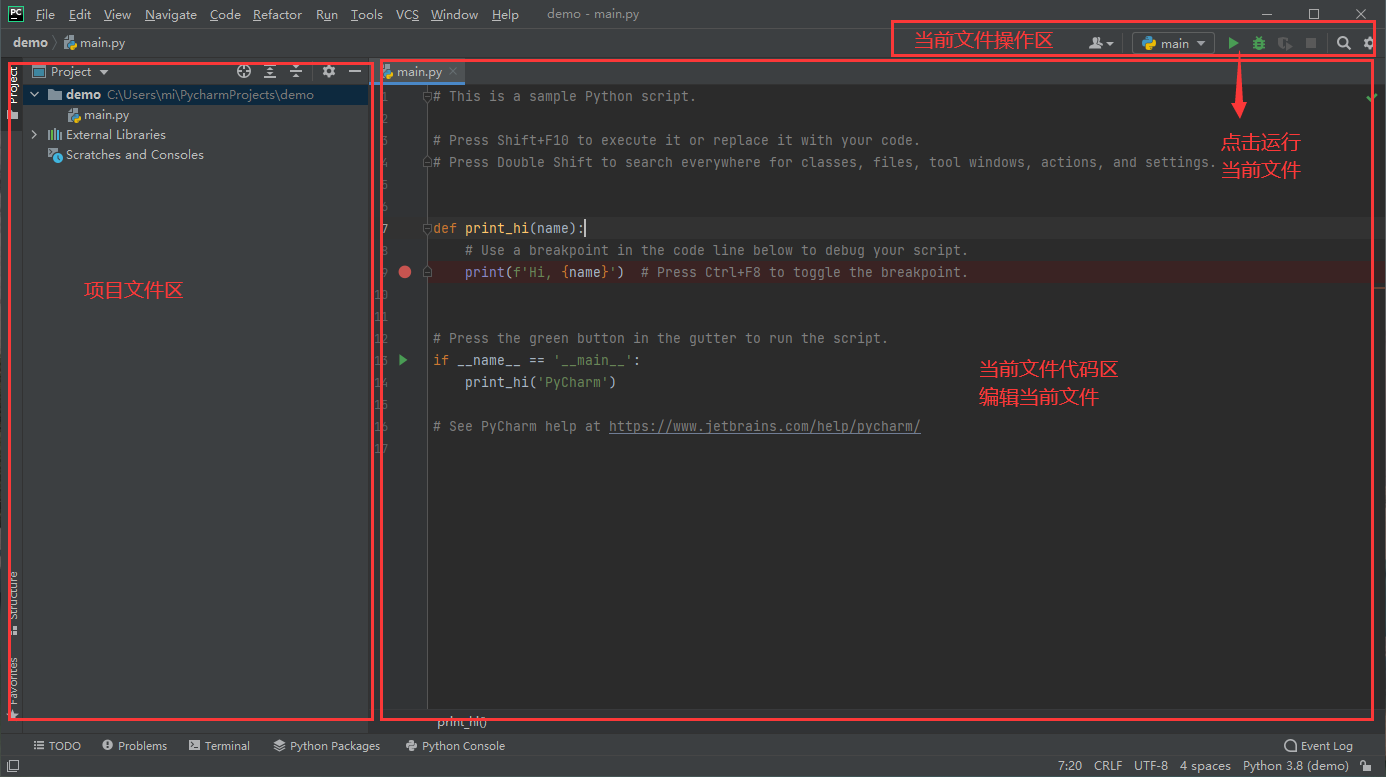（1）直接点击PyCharm操作区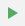按钮

（2）在文件编辑区单击鼠标右键，选择Run…选项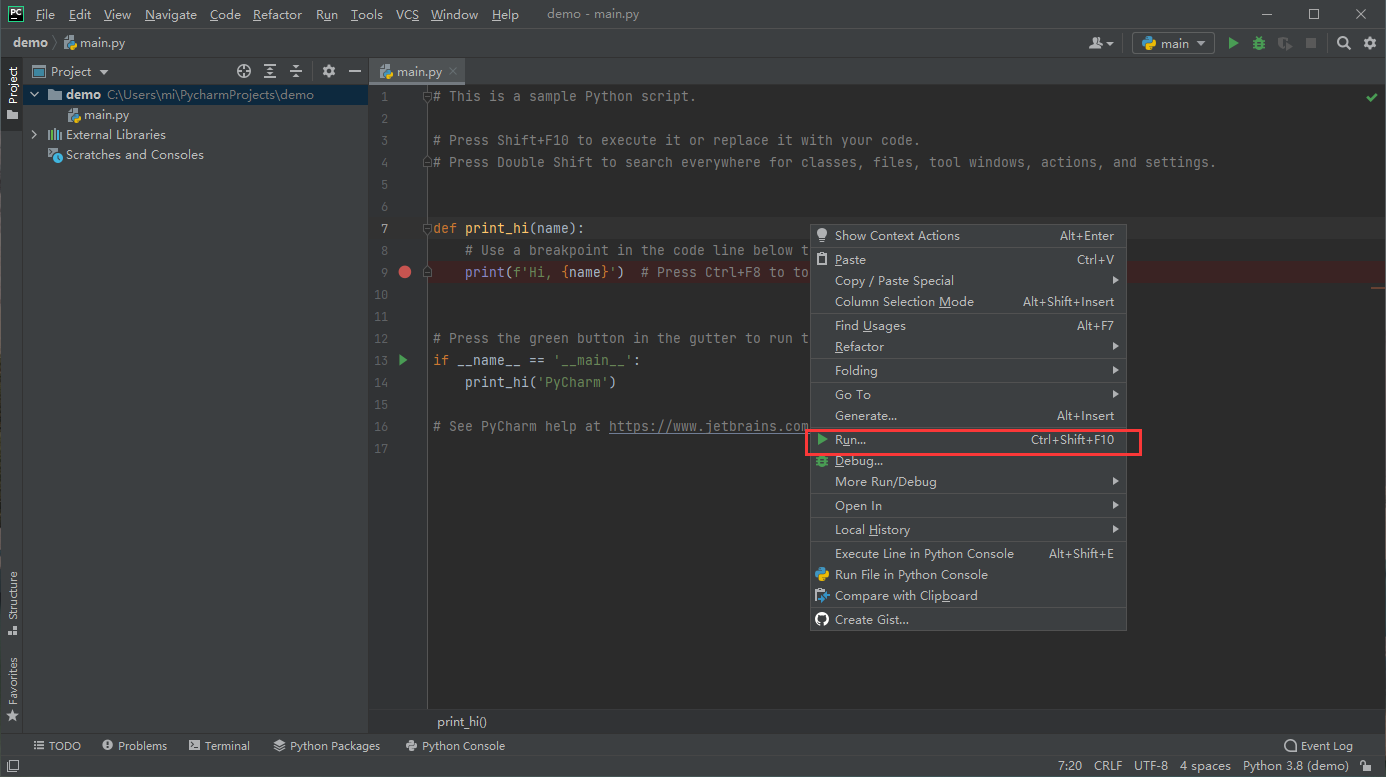（3）在Terminal运行文件夹下输入：

``````python demo1.py
``````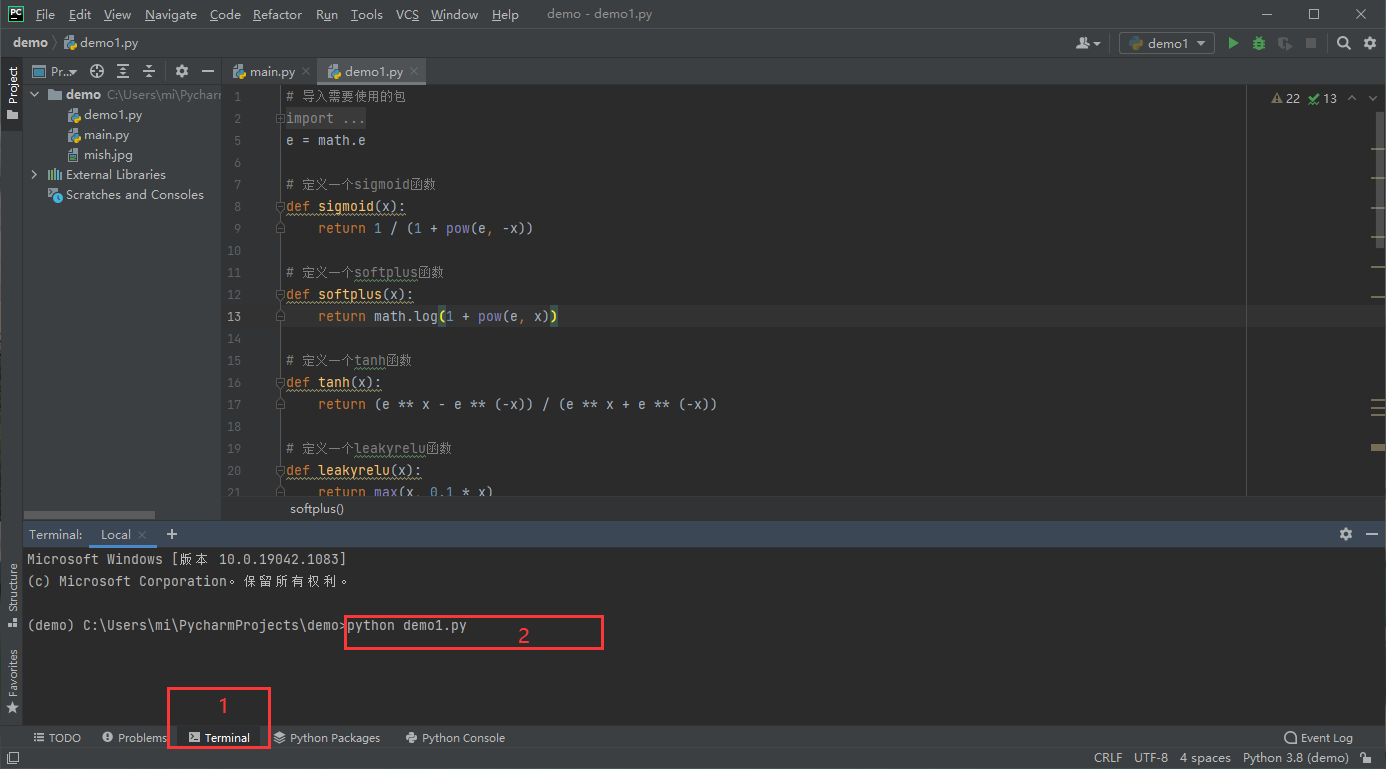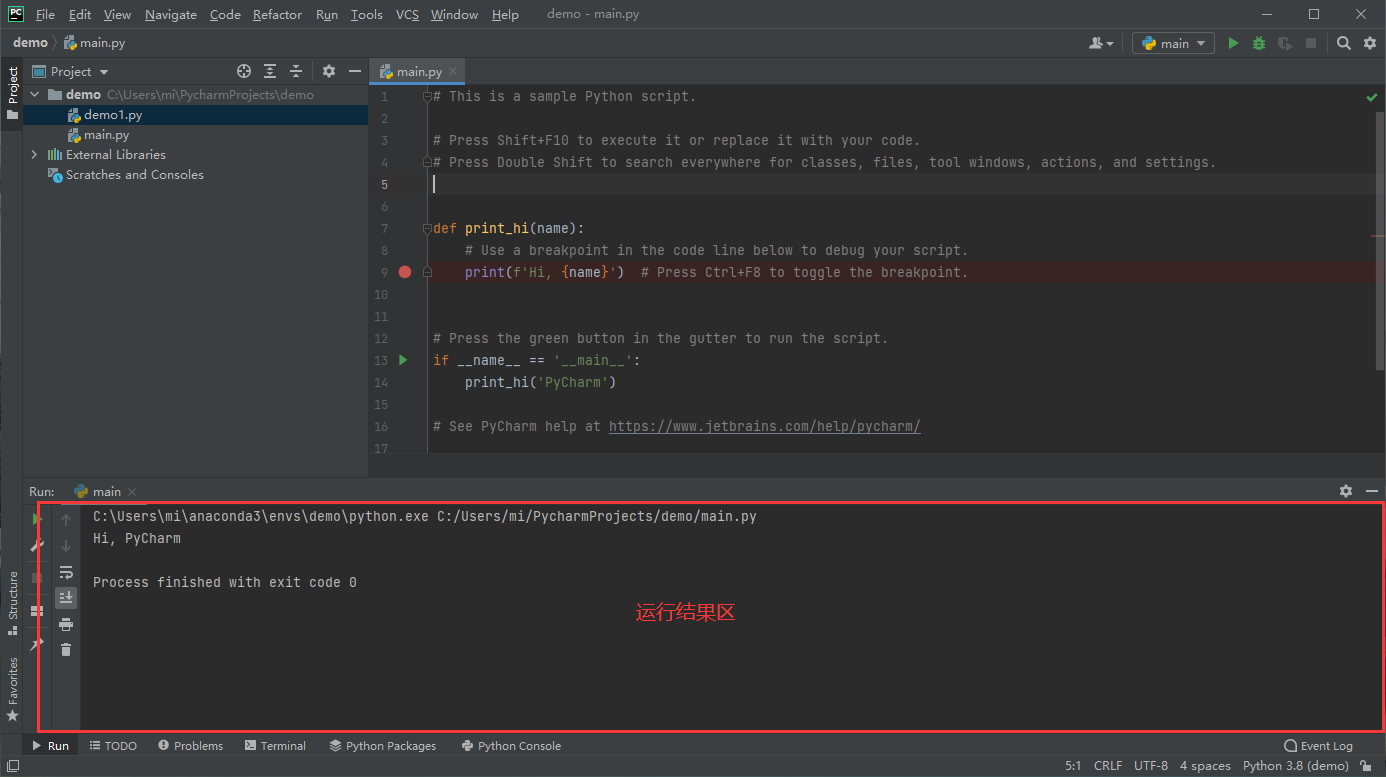1. 新建文件，在项目文件区demo文件夹单击鼠标右键，按照下图所示选择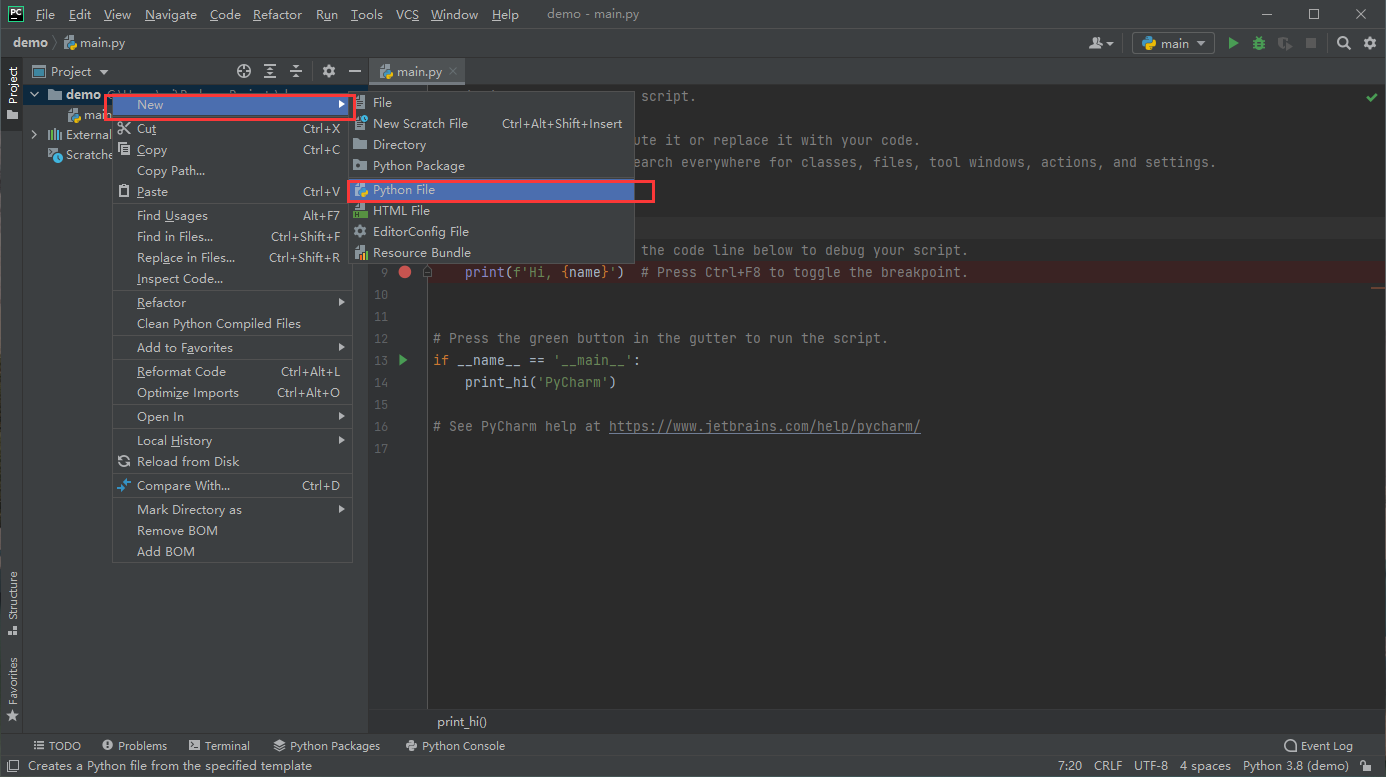``````# 导入需要使用的包
import numpy as np
import math
import matplotlib.pyplot as plt
e = math.e

# 定义一个sigmoid函数
def sigmoid(x):
return 1 / (1 + pow(e, -x))

# 定义一个softplus函数
def softplus(x):
return math.log(1 + pow(e, x))

# 定义一个tanh函数
def tanh(x):
return (e ** x - e ** (-x)) / (e ** x + e ** (-x))

# 定义一个leakyrelu函数
def leakyrelu(x):
return max(x, 0.1 * x)

# 定义一个mish函数
def mish(x):
return x * tanh(softplus(x))

# 限定x,y坐标轴范围为(-5,5),并在其中产生1000个相应的点
x = np.linspace(-5, 5, 1000)
y0 = np.linspace(-5, 5, 1000)
y1 = np.linspace(-5, 5, 1000)
y2 = np.linspace(-5, 5, 1000)
# 将函数值赋值给上述产生的点
for i in range(1000):
y0[i] = sigmoid(x[i])
y1[i] = leakyrelu(x[i])
y2[i] = mish(x[i])
# 绘制函数图像
plt.plot(x, y0, color='blue', linewidth=1, label='sigmoid')
plt.plot(x, y1, color='red', linewidth=1, label='leakyrelu')
plt.plot(x, y2, color='orange', linewidth=1, label='mish')
plt.title('sigmoid, leakyrelu and Mish functions', fontdict={'family': 'Times New Roman', 'size': 14})
plt.xticks(fontproperties = 'Times New Roman', size = 14)
plt.legend(prop={'family' : 'SimSun', 'size' : 14})
plt.yticks(fontproperties = 'Times New Roman', size = 14)
plt.grid()
plt.savefig('mish.jpg', dpi=600, bbox_inches='tight')
plt.show()
``````
1. 安装Python包。在numpy和matplotlib下有红线，表示相应的包未安装。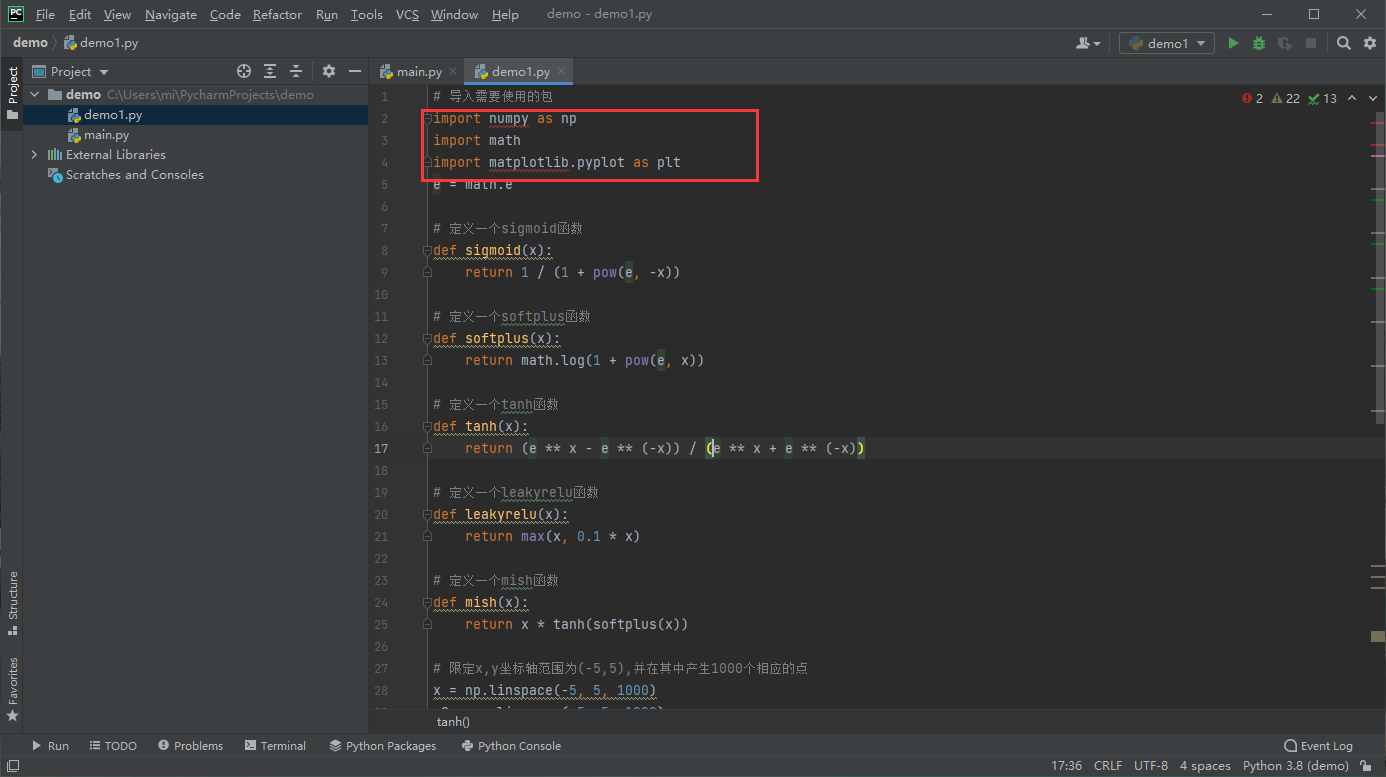``````pip install numpy
``````

``````pip install numpy -i https://pypi.tuna.tsinghua.edu.cn/simple
``````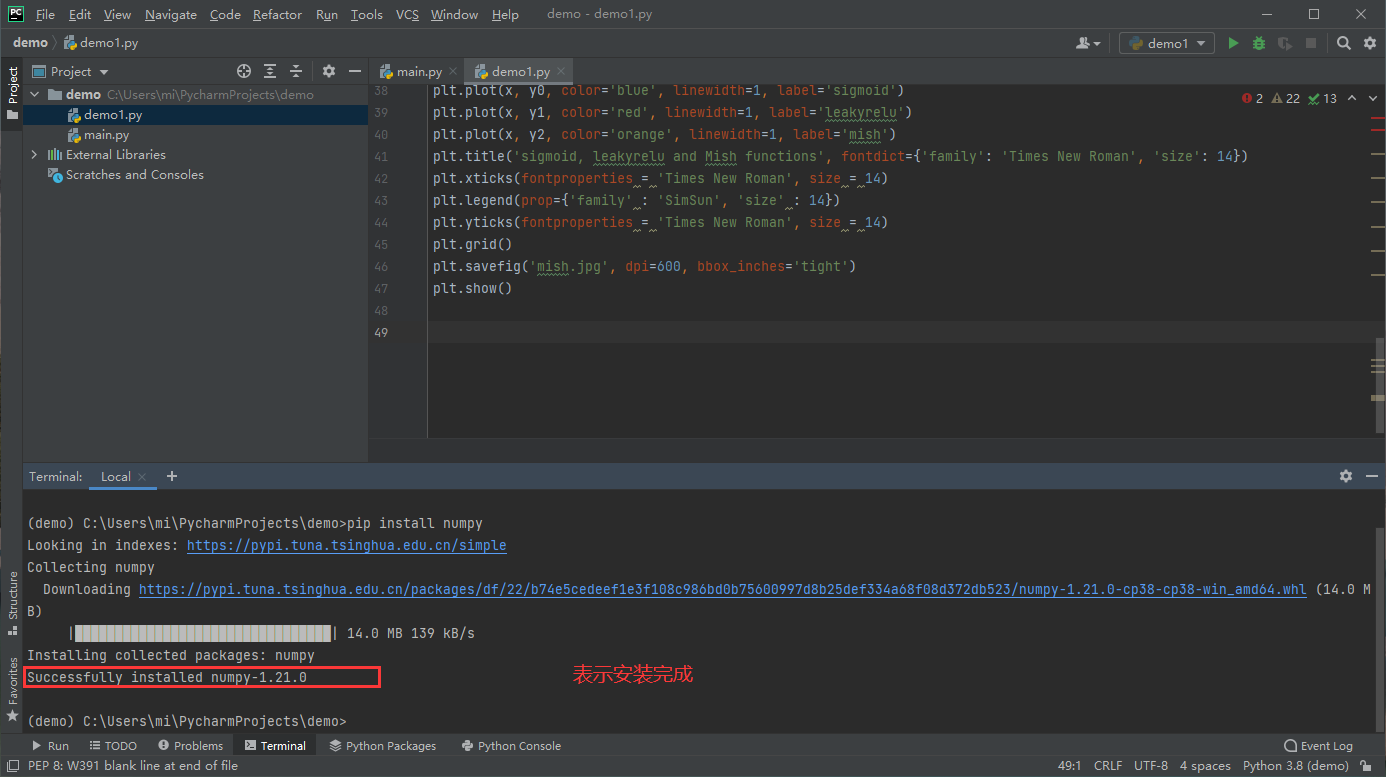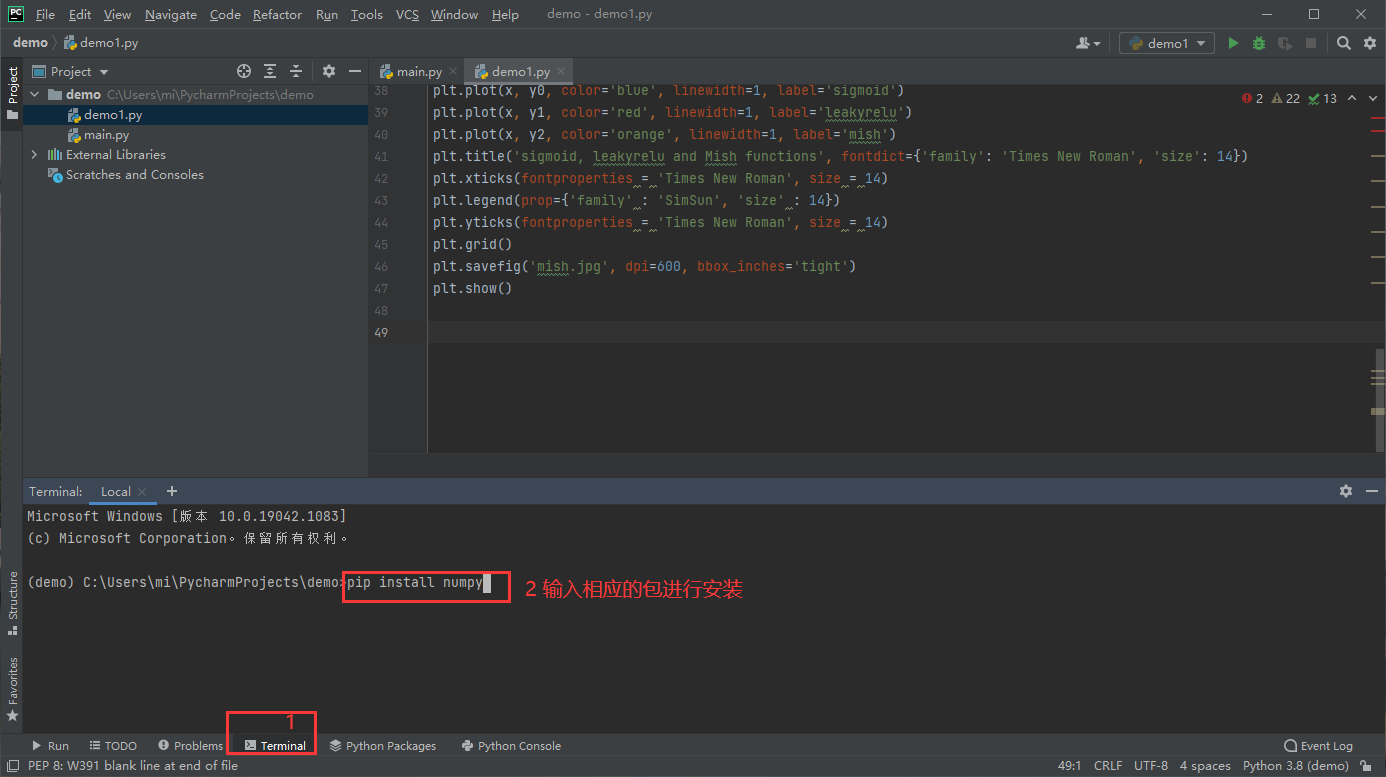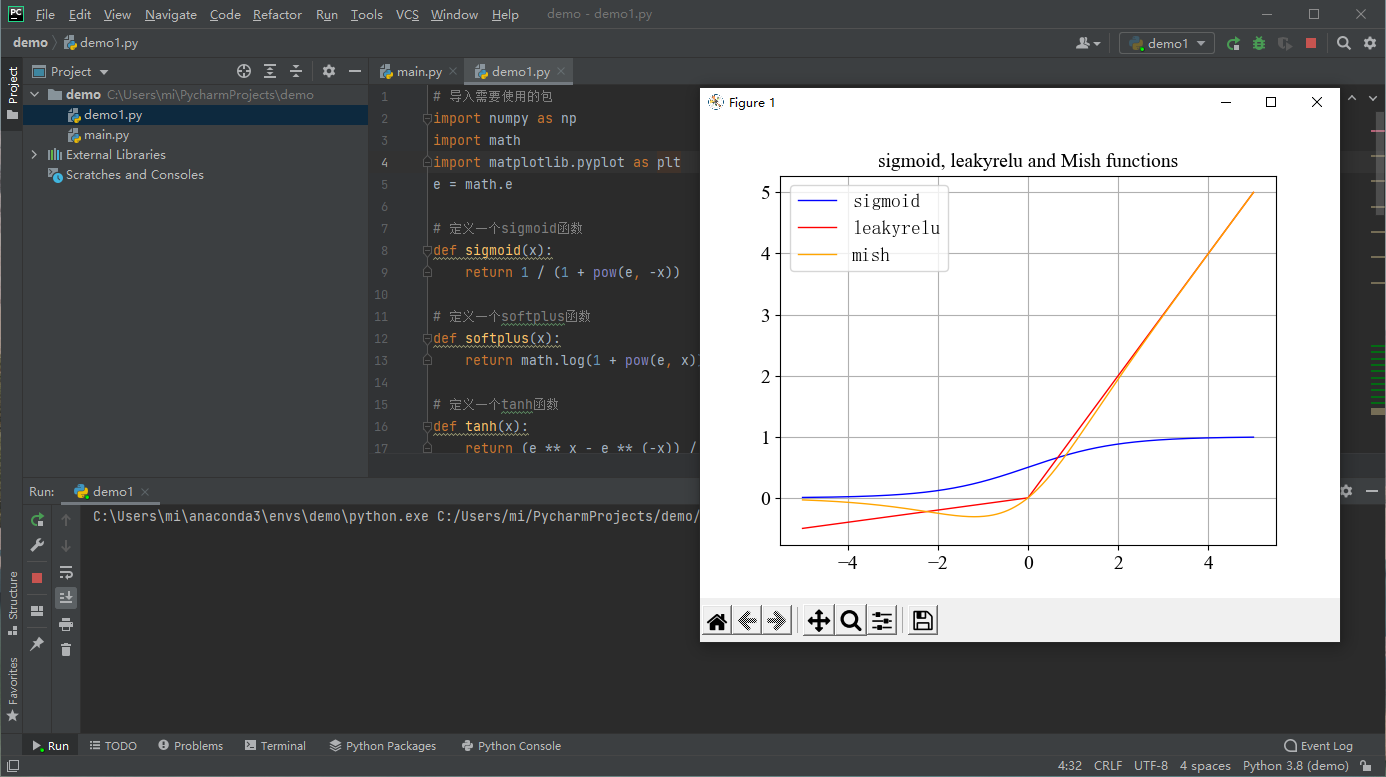# 五、 安装jupyter notebook

1. 打开anaconda

2. 激活我们建的环境demo，输入：

``````conda activate demo
``````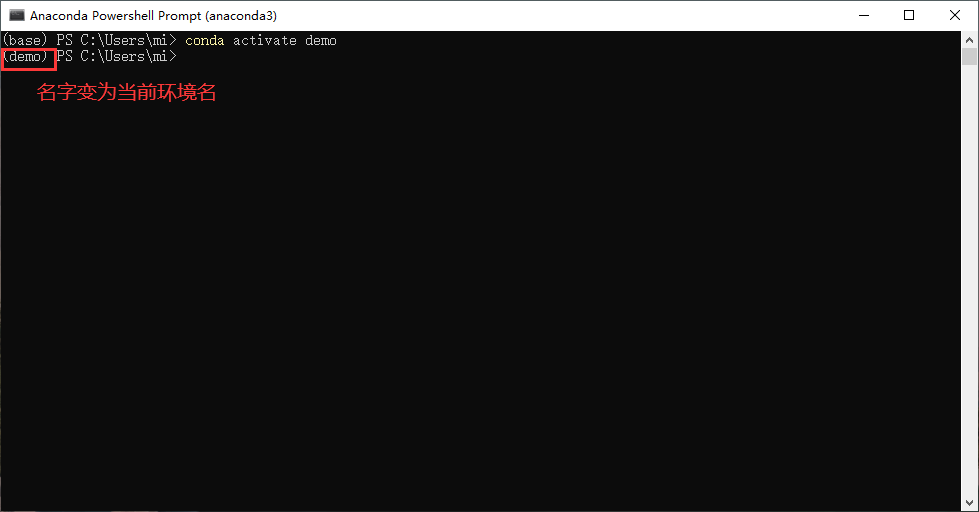1. 安装jupyter notebook ，输入：

``````pip install jupyter notebook
``````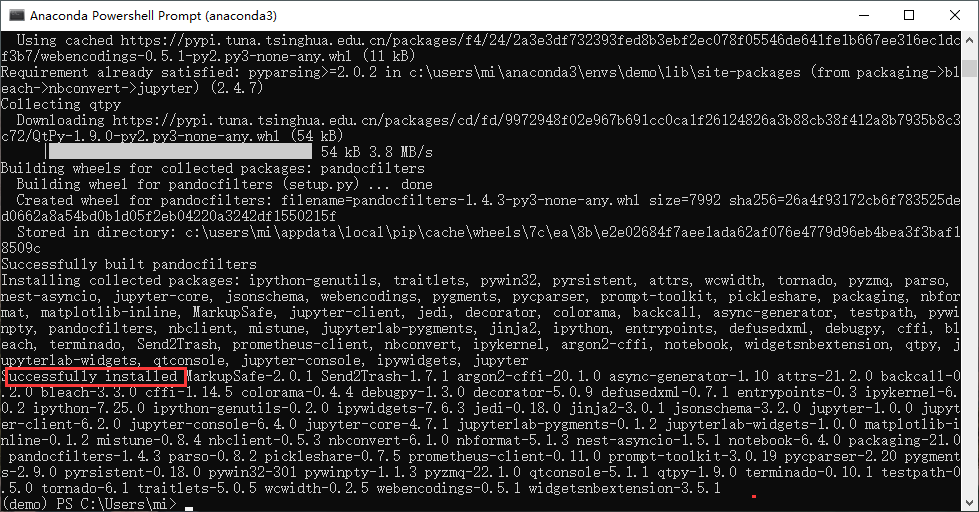jupyter notebook安装完成

1. 打开jupyter notebook，输入：

``````jupyter notebook
``````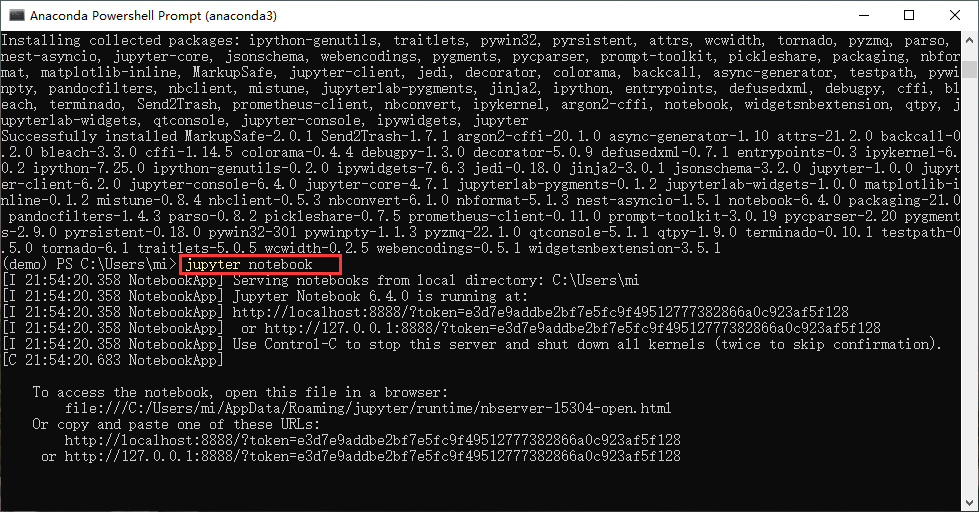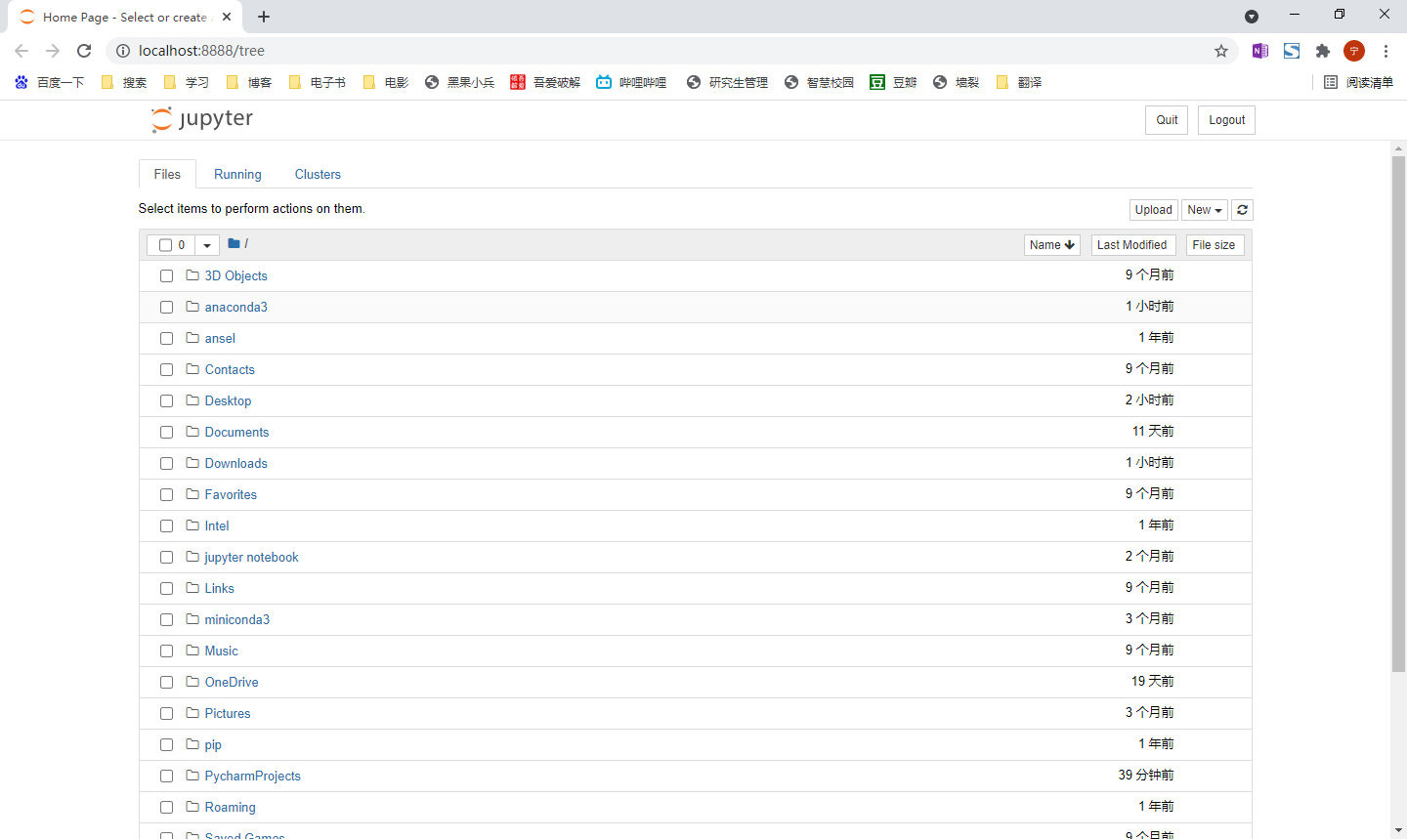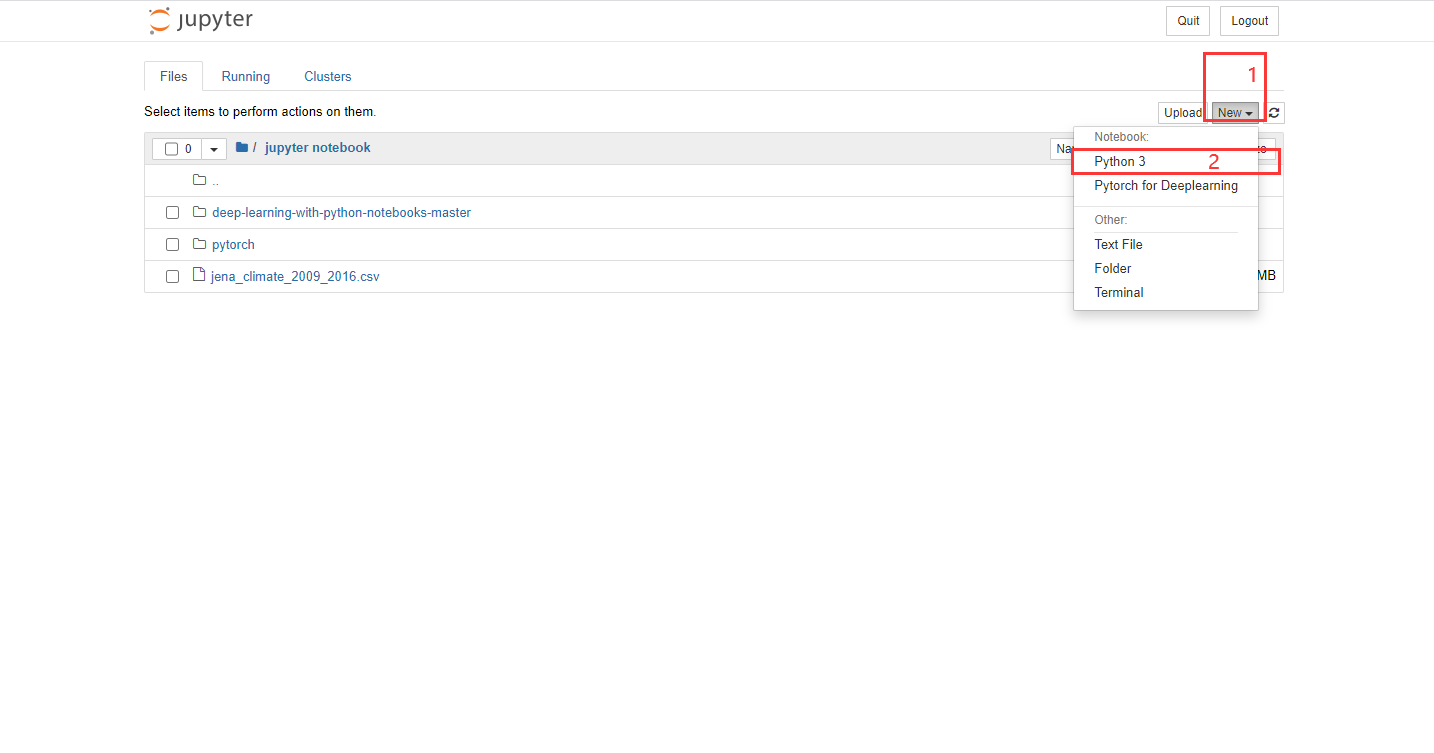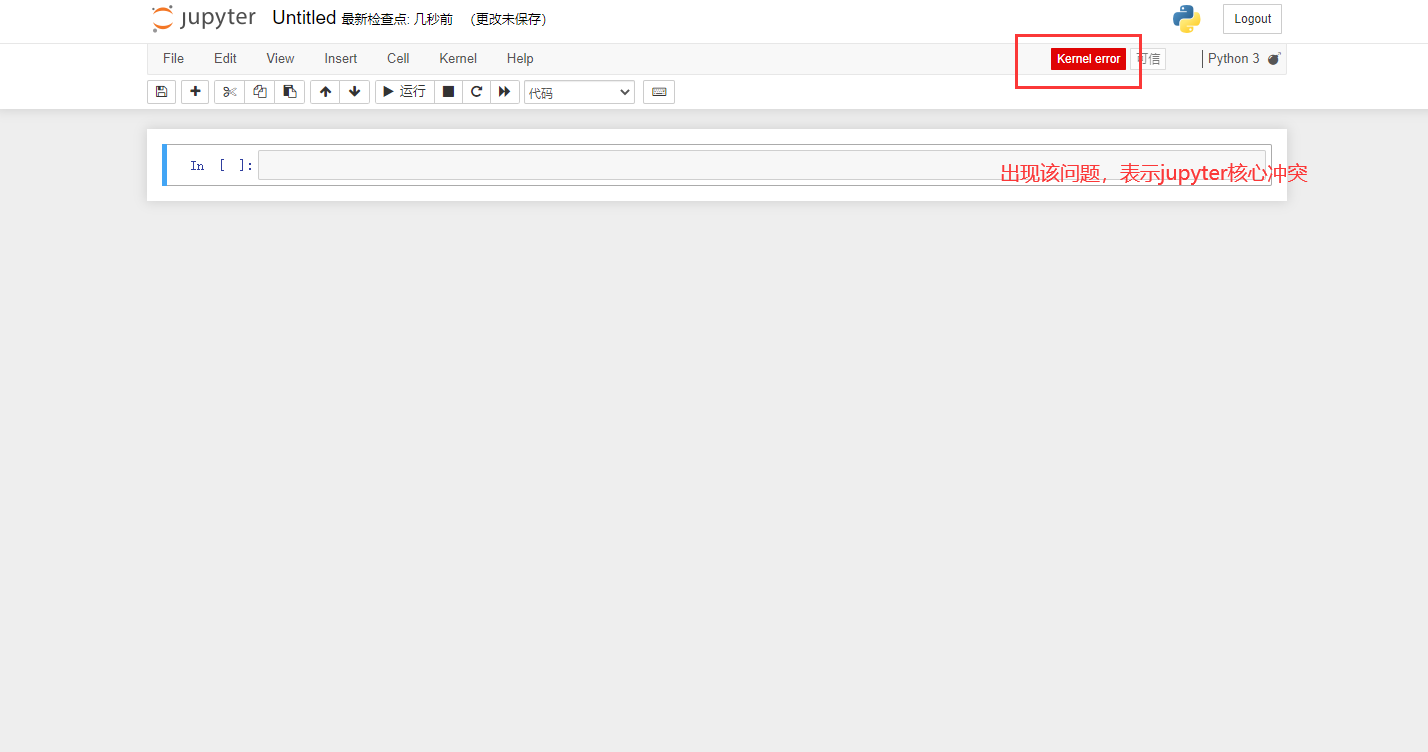（1） 在刚才打开的Anaconda页面按键盘 Ctrl+C 退出当前jupyter程序。

（2） 卸载pywin32, 输入：

``````pip uninstall pywin32
``````

（3） 安装225版本pywin32, 输入：

``````pip install pywin32==225
``````

（1） 打开Anaconda

（2） 输入：

``````conda create demo
``````

（3） 输入：

``````jupyter notebook
``````
1. 运行第一个jupyter程序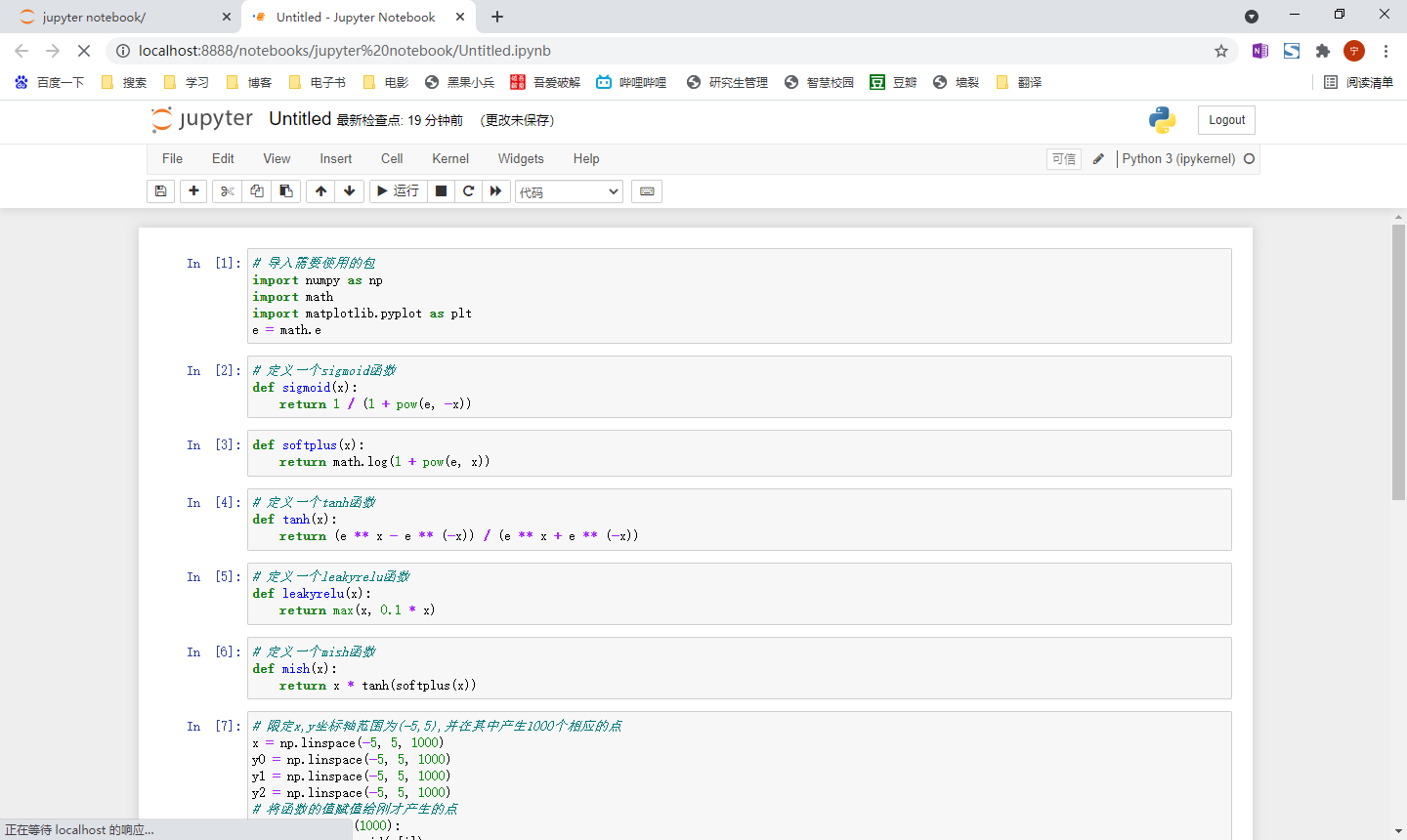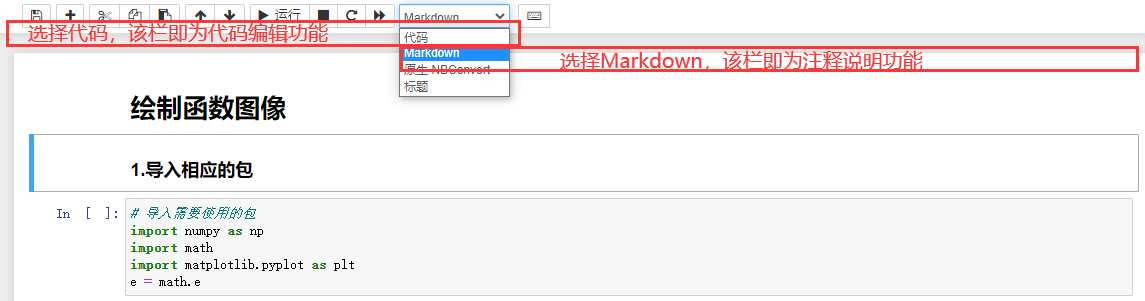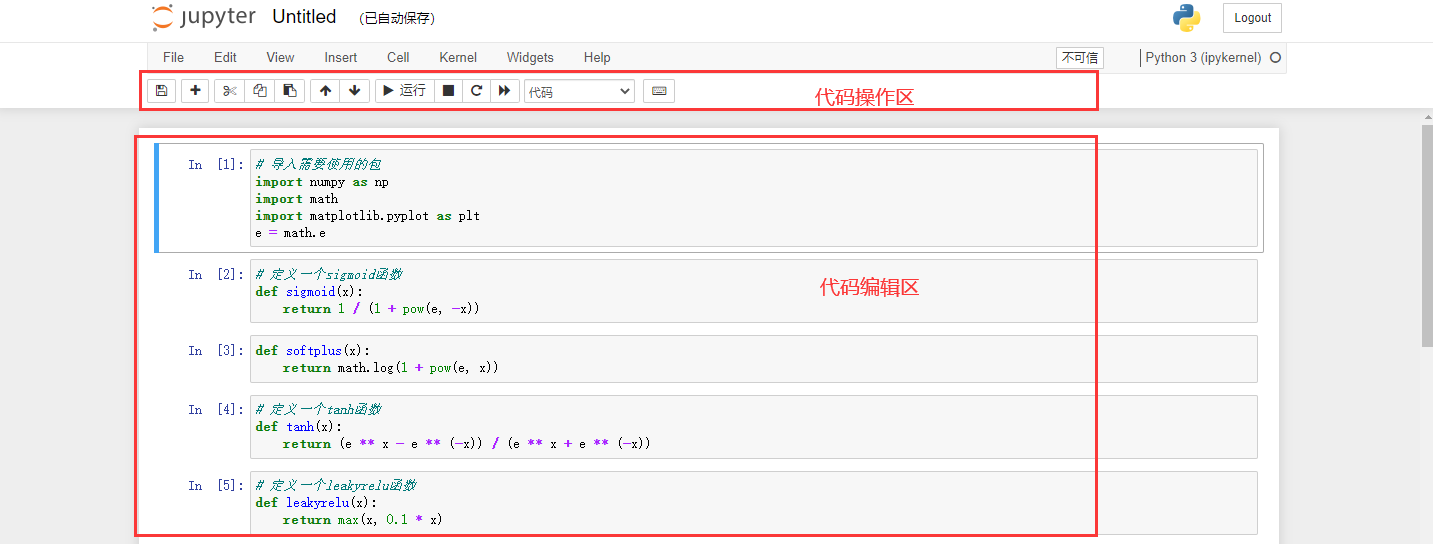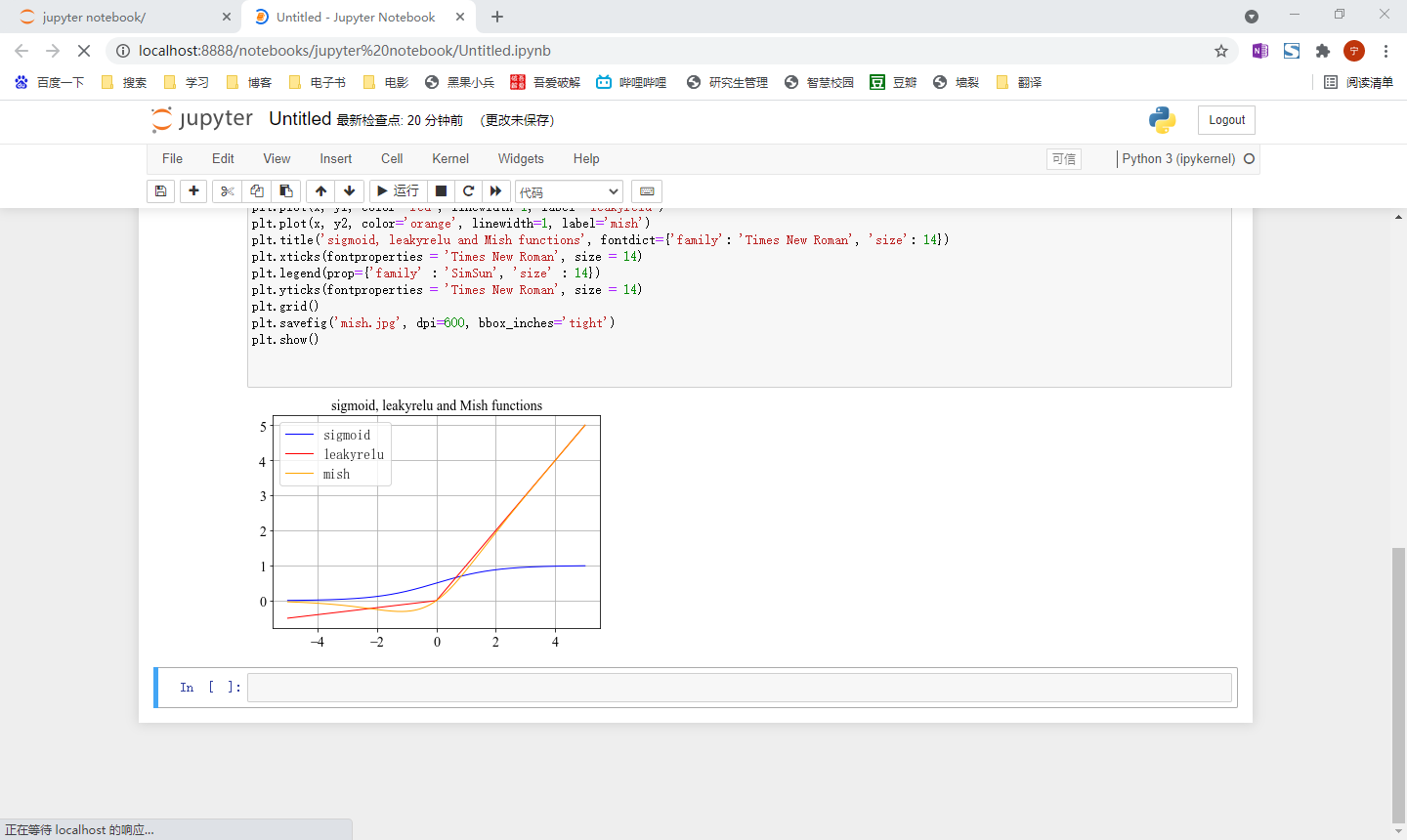Jupyter notebook的强大之处在于，可以对代码段的结果进行输出。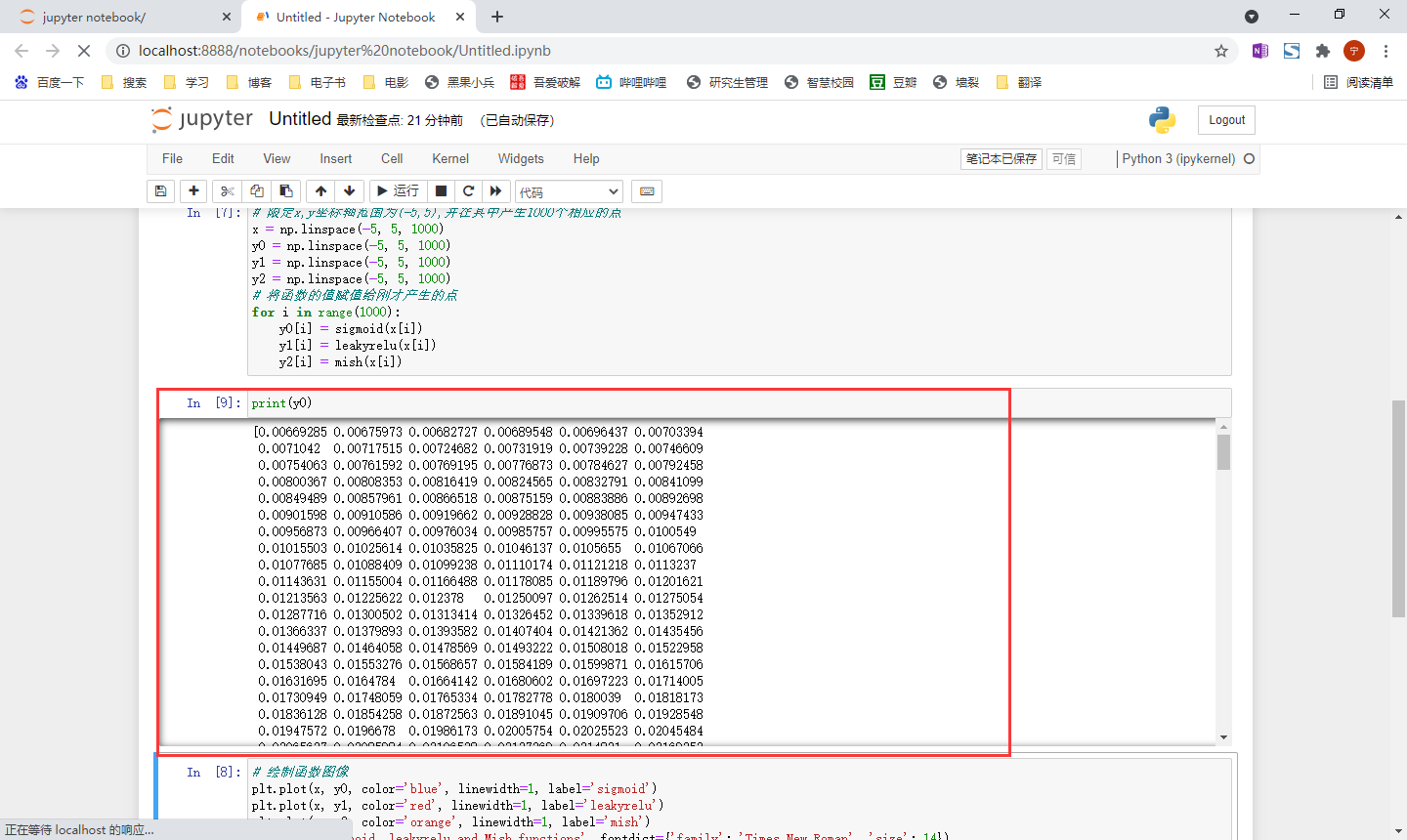1. Jupyter安装python包。

（1） 打开Anaconda

（2） 激活当前环境，输入：

``````conda activate demo
``````

（3） 安装相应的包，输入：

``````pip install 包名
``````

# 附录

## 1. PyCharm字体大小，主题设置

（1）调节主题颜色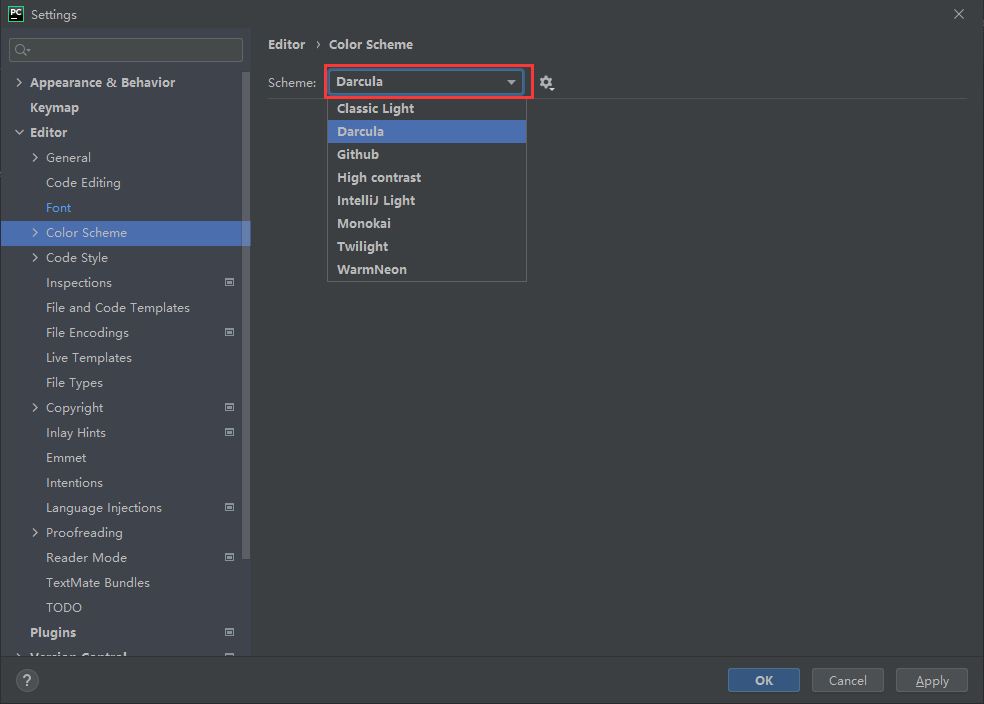（2）调节字体大小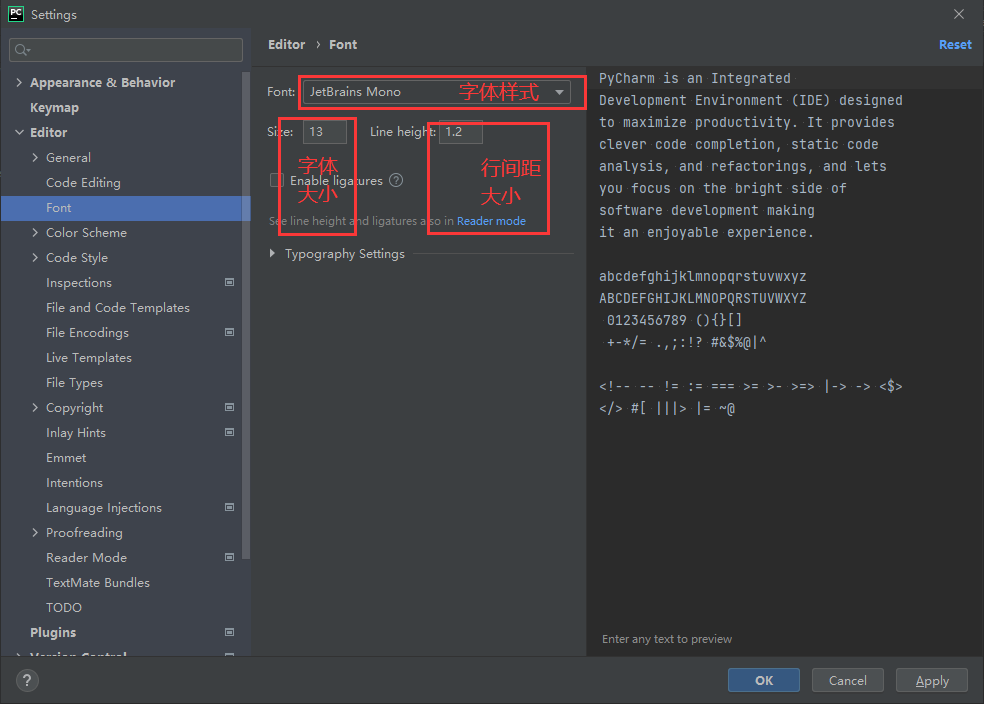## 2. Anaconda常用命令

``````conda create -n demo python=3.8	  创建一个名字为demo的虚拟环境，python版本为3.8
conda info -e			          查看虚拟环境列表
conda activate demo			      Windows系统激活虚拟环境demo
source activate demo              Mac & Linux 系统激活虚拟环境demo
conda install numpy 		      使用conda安装numpy包
conda install numpy==1.11.0 	  使用conda安装版本指定为1.11.0的numpy包
conda uninstall numpy 		      使用conda卸载numpy包
conda list				          查看已安装包列表
conda remove --name demo –all     删除demo虚拟环境
pip list 				          查看已安装包列表
pip install numpy			      使用pip安装numpy包
pip install numpy==1.11.0 		  使用pip安装版本指定为1.11.0的numpy包
pip install numpy -i	https://pypi.tuna.tsinghua.edu.cn/simple        使用pip清华镜像源安装numpy包
pip uninstall numpy			      使用pip卸载numpy包
``````